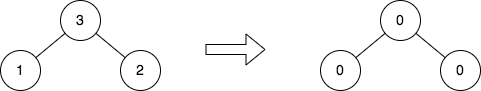2641. Cousins in Binary Tree II
Medium
311
3

Given the `root` of a binary tree, replace the value of each node in the tree with the sum of all its cousins' values.

Two nodes of a binary tree are cousins if they have the same depth with different parents.

Return the `root` of the modified tree.

Note that the depth of a node is the number of edges in the path from the root node to it.

Example 1:```Input: root = [5,4,9,1,10,null,7]
Output: [0,0,0,7,7,null,11]
Explanation: The diagram above shows the initial binary tree and the binary tree after changing the value of each node.
- Node with value 5 does not have any cousins so its sum is 0.
- Node with value 4 does not have any cousins so its sum is 0.
- Node with value 9 does not have any cousins so its sum is 0.
- Node with value 1 has a cousin with value 7 so its sum is 7.
- Node with value 10 has a cousin with value 7 so its sum is 7.
- Node with value 7 has cousins with values 1 and 10 so its sum is 11.
```

Example 2:```Input: root = [3,1,2]
Output: [0,0,0]
Explanation: The diagram above shows the initial binary tree and the binary tree after changing the value of each node.
- Node with value 3 does not have any cousins so its sum is 0.
- Node with value 1 does not have any cousins so its sum is 0.
- Node with value 2 does not have any cousins so its sum is 0.
```

Constraints:

• The number of nodes in the tree is in the range `[1, 105]`.
• `1 <= Node.val <= 104`
Accepted
11.2K
Submissions
16.3K
Acceptance Rate
68.6%

Seen this question in a real interview before?
1/4
Yes
No

Discussion (0)

Related Topics